# ISEE Upper Level Quantitative : Other Polygons

## Example Questions

← Previous 1

### Example Question #1 : Other Polygons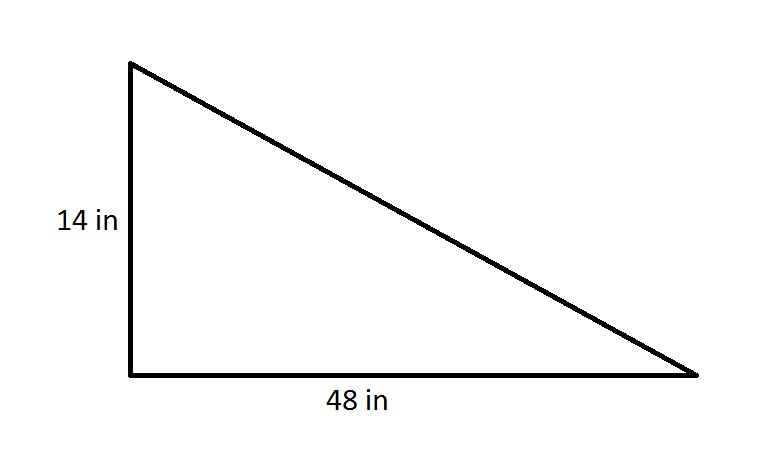The length of a side of a regular octagon is one and a half times the hypotenuse of the above right triangle. Give the perimeter of the octagon in feet.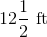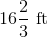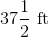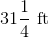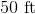Explanation:

By the Pythagorean Theorem, the hypotenuse of the right triangle is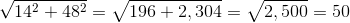inches.

The sidelength of the octagon is therefore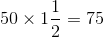inches,

and the perimeter of the regular octagon, which has eight sides of equal length, is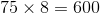inches,

or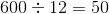feet.

### Example Question #2 : Other Polygons

An equilateral triangle, a square, a regular pentagon, a regular hexagon, and a regular octagon have the same sidelength. Which is the greater quantity?

(A) The median of their perimeters

(B) The midrange of their perimeters

(A) and (B) are equal

(B) is greater

It is impossible to tell which is greater from the information given

(A) is greater

(B) is greater

Explanation:

The answer is independent of the sidelength, so we can assume without loss of generality that the sidelength is 1. The equilateral triangle, the square, the pentagon, the hexagon, and the octagon have 3, 4, 5, 6, and 8 sides of equal length, respectively, so their perimeters are 3, 4, 5, 6, and 8.

The median of these perimeters is the middle perimeter, 5. The midrange of these perimeters is the mean of the greatest and the least perimeters: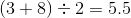The midrange, (B), is greater.

### Example Question #3 : Other Polygons

A square, a regular pentagon, a regular hexagon, and a regular octagon have the same sidelength. Which is the greater quantity?

(A) The mean of their perimeters

(B) The median of their perimeters

(A) and (B) are equal

It is impossible to tell which is greater from the information given

(A) is greater

(B) is greater

(A) is greater

Explanation:

The answer is independent of the sidelength, so we can assume without loss of generality that the sidelength is 1. The square, the pentagon, the hexagon, and the octagon have 4, 5, 6, and 8 sides of equal length, respectively, so their perimeters are 4, 5, 6, and 8. The mean of these four perimeters is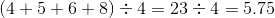units.

The median is the mean of the middle two perimeters, which are 5 and 6: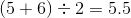The mean, (A), is greater.

### Example Question #4 : Other Polygons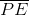is a side of regular Pentagon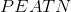as well as Square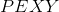, which is completely outside Pentagon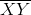is a side of equilateral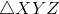, where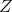is a point outside Square. Which is the greater quantity?

(a) The perimeter of Pentagon(b) The perimeter of Pentagon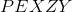(a) and (b) are equal

It is impossible to determine which is greater from the information given

(a) is the greater quantity

(b) is the greater quantity

(a) and (b) are equal

Explanation:

The figure referenced is below: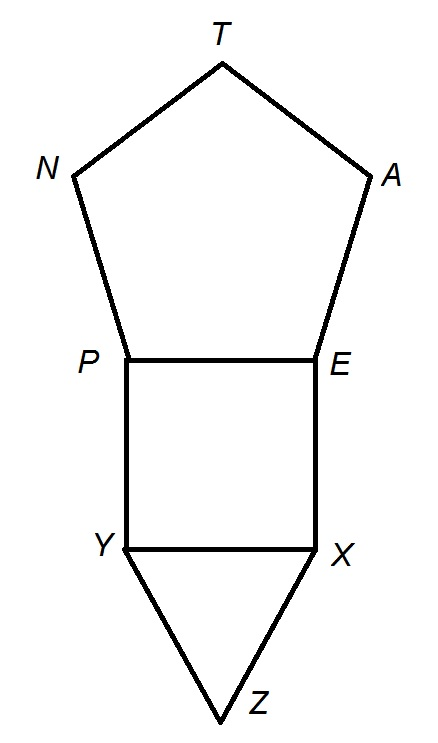Pentagonis regular, so all of its sides have the same length; we will examinein particular. The perimeter of Pentagonis the sum of the lengths of its sides, which is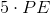.

Sinceis also a side of Square, it follows that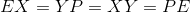; sinceis also a side of equilateral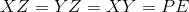. The perimeter of Pentagonis equal to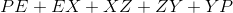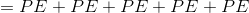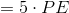,

the same as that of Pentagon.

### Example Question #5 : Other PolygonsA regular decagon has the same perimeter as the above right triangle. Give the length of one side.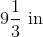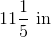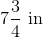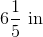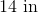Explanation:

By the Pythagorean Theorem, the hypotenuse of the right triangle isinches, making its perimeter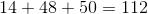inches.

A regular decagon has ten sides of equal length, so each side measures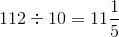inches.

### Example Question #6 : Other Polygons

A regular octagon has perimeter one meter. Which is the greater quantity?

(A) The length of one side

(B) 125 millimeters

(B) is greater

It is impossible to determine which is greater from the information given

(A) and (B) are equal

(A) is greater

(A) and (B) are equal

Explanation:

A regular octagon has eight sides of equal length. The perimeter of this octagon is one meter, which is equal to 1,000 millimeters; each side, therefore, has length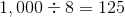millimeters

making the quantities equal.

### Example Question #7 : Other Polygons

A regular pentagon has sidelength 72; the perimeter of a regular hexagon is 80% of that of the pentagon. Which is the greater quantity?

(A) The length of one side of the hexagon

(B) 50

It is impossible to determine which is greater from the information given

(B) is greater

(A) is greater

(A) and (B) are equal

(B) is greater

Explanation:

A regular pentagon has five sides of equal length; since one side is 72 units long, its perimeter is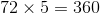.

80% of this is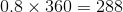,

so this is the length of the hexagon, and, since all six sides are of equal length, one side measures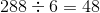(B) is greater.

### Example Question #8 : Other Polygons

A regular octagon has twice the perimeter of a regular pentagon. What is the ratio of the sidelength of the octagon to that of the pentagon?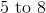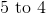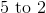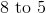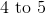Explanation:

The solution is independent of the actual lengths, so we assume the pentagon has sidelength 1. Its perimeter is therefore 5. Subsequently, the octagon's perimeter is twice this, or 10, and its sidelength is one-eighth of this, or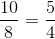.

The ratio of the sidelength of the octagon to that of the pentagon is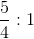or 5 to 4.

### Example Question #1 : How To Find The Length Of A Side

A regular octagon has perimeter one mile.Which is the greater quantity?

(a) The length of one side

(b) 880 feet

(b) is the greater quantity

(a) is the greater quantity

It is impossible to determine which is greater from the information given

(a) and (b) are equal

(b) is the greater quantity

Explanation:

A regular octagon has eight sides of equal length. The perimeter, which is the sum of the lengths of these sides, is one mile, which is equal to 5,280 feet. Therefore, the length of one side is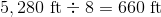. This makes the length of a side less than 880 feet.

### Example Question #10 : Other Polygons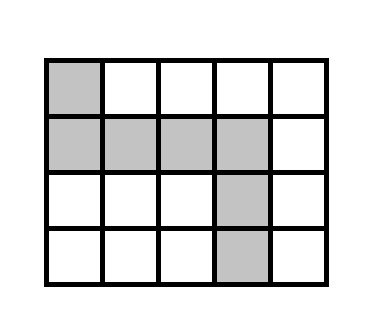The above rectangle, which has perimeter 360, is divided into squares of equal size. Give the area of the shaded portion.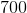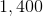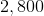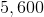Explanation:

The sides of the rectangle, in total, are divided into 18 segments of equal measure, as indicated below: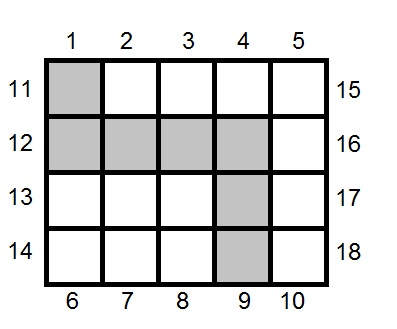The rectangle has a total perimeter of 360, so each segment - one side of a square - measures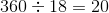. Each square has area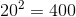, so the shaded portion of the rectangle, which comprises seven squares, has area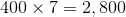.

← Previous 1